# Introduction

You can solve a system of linear equation using Gaussian elimination and backward substitution, however there are two drawbacks of using such a technique: the Gaussian elimination component of the algorithm is slow, O(n3), relative to the backward substitution component, O(n2), and the Gaussian elimination is subject to numeric problems.

In this topic, we will see how we can avoid doing Gaussian elimination repeatedly and thereby perform the most expensive component of the algorithm only once, using the faster O(n2) operations to solve numerous different problems.

# Background

Useful background for this topic includes:

• Gaussian elimination and backward-substitution.

# Interactive Maplet

A PLU Decomposition Tutorial

# Solving Systems of Linear Equations

Consider the following system of n equations and n unknowns.

m1,1 x1 + m1,2 x2 + ⋅⋅⋅ m1,n xn = b1
m2,1 x1 + m2,2 x2 + ⋅⋅⋅ m2,n xn = b2

mn,1 x1 + mn,2 x2 + ⋅⋅⋅ mn,n xn = bn

In linear algebra, you saw how you could convert this into a matrix-vector problem

M x = b

where M = (mi,j) is the matrix of coefficients, x = (xi)T is a column vector of unknowns and b = (bi)T is a column vector of known values.

To solve such a system, you would create the augmented matrix (M|b) and proceed with applying Gaussian elimination and backward substitution.

If you do not recall forward or backward substitution, please see the corresponding HOWTO pages (follow the links).

# Problems with Gaussian Elimination

There are two problems with Gaussian elimination:

## 1. Speed

Even if you do an optimal implementation of Gaussian elimination and backward substitution for solving a system of n linear equations, you require the following number of operations:

Table 1. Operations due to Gaussian elimination.

• n2/2 − n/2 divisions and negations
• n3/3 − n/3 multiplications and additions

Table 2. Operations due to backward substitution.

• n2/2 − n/2 multiplications and subtractions
• n divisions

Thus, most of the time is spent in Gaussian elimination: for example, for a 1000 × 1000 matrix M would require 333832500 operations to do the Gaussian elimination, but only 500500 operations to do the backward substitution. This is summarized by stating that Gaussian elimination is O(n3) while backward substitution (and forward substitution, for that matter) are O(n2).

## 2. Numeric Instability

Try solving the following system of equations using 3 decimal digits of precision:

Performing Gaussian elimination, we add -21.4/0.353 = -60.6 times row 1 onto row 2 to get:

which is now in echelon form, so we may perform backward substitution:

• x2 = 0.125
• x1 = 0

If we now try to solve this using Matlab, we get a very different answer, namely x = (-0.01280, 0.12536)T. Our solution for x2 may be reasonable (a relative error of 0.0029), but the relative error of 0 for any number is 1.0, so this is a very poor approximation.

Try it again, only now swap the two rows:

and apply Gaussian elimination:

Applying backward substitution yields x2 = 0.126 and x1 = -0.0133. Thus, the relative error of both of these numbers are 0.0051 and 0.039, respectively: a significant overall improvement.

What happened here was that by adding a large multiple of the first row onto the second, because of the finite precision of our approximation, we ended up with a matrix of the form

and when we apply backward substitution, we get x2 = b1/m12 and therefore x1 = 0.

# Solving Problem 1

Suppose we could write the matrix M as a product of two matrices: M = L U where L is a lower-triangular matrix and U is an upper-triangular matrix. In this case, we could then rewrite the matrix-vector problem M x as

L U x = b

Thus, let us define y = U x. In this case, the equation may be written as:

L y = b

In this case, L is a lower-triangular matrix and therefore we do not have to perform Gaussian elimination to solve for y and instead we only use forward substitution. Similarly, once we have found y, we may now solve:

U x = y

for x. Again, U is upper-triangular, so we again do not have to perform Gaussian elimination, we need only use backward substitution.

From before we may now calculate the number of required operations to perform both forward and backward substitution:

Table 3. Operations due to forward and backward substitution.

• n2n multiplications and subtractions
• 2n divisions

Thus, if we can find L and U, we could easily solve M x = bi for N different known vectors bi using only Nn2 + Nn operations.

Fortunately, we are already half way to writing M as a product L U: when we do Gaussian elimination, we are left with an upper-triangular matrix U. By looking at how we got to finding U, we will see that we can, simultaneously, find the corresponding lower-triangular matrix L.

First, recall that adding a multiple of one row onto another row of a matrix is termed an elementary operation and may be represented by an elementary matrix. If you are adding c times row ν onto row μ, the corresponding elementary matrix E = (ei j) is an n × n identity matrix with the entry eμ ν = c.

Consider the following example of a Gaussian elimination:

Adding -0.5 times row 1 onto row 2 yields:

Adding -0.2 times row 1 onto row 3 yields:

Finally, adding 0.1 times row 2 onto row 3 yields

Now, the inverse of the elementary matrices defined above are simply the identity matrix with the negative of the off-diagonal entry, so by multiplying on the left by each of the three inverses, we get:

If you multiply out the three elementary matrices in the given order, you will find that the product is simply:

Thus, we have found an LU decomposition of the matrix M. It should be noted that there are many LU decompositions.

Thus, if we wished to solve M x = b where b = (-19., -1.5, -28.6)T, we would apply forward substitution to solve L y = b to get that y = (-19, 8, -24)T and then solve U x = y using backward substitution to find that x = (-2, 2, 3)T.

Therefore, we only have to solve the LU decomposition once but we can solve hundreds of systems of equations of the form M x = bi at a much cheaper cost.

# Solving Problem 2

In the above example, we solved the first problem of time: solve once for L and U and then use these to solve for many solution vectors using the much less expensive forward and backward substitutions. However, we did not solve the second problem, that of the numerical instability.

If we examine the problem, we note that it occurs because we are adding a large multiple of one row onto another. Recall that with Gaussian elimination, if you found a zero on the diagonal, you could swap the row containing the diagonal with a row beneath it which did not contain a zero in that column. We will do the same thing here, but instead, with each step, we will first swap the row with largest element (in absolute value) on or below the diagonal with the row containing the diagonal. The only thing left to do is to keep track of the row swaps.

This may be done by factoring the matrix M as a triplet P L U where P is a permutation matrix. As the inverse of a permutation matrix is simply its transpose, if we try to solve P L U x = b, it is equivalent to solving L U x = PTb.

Without proof, the HOWTO for this section covers how to find the three matrices PT, L and U in a reasonably efficient and straight-forward manner.

# Problem

Given the n × n matrix M, find a decomposition M = PLU where P is a permutation matrix, L is a lower-triangular matrix, and U is an upper-triangular matrix. Next, given a column vector b, solve the system of linear equations defined by M x = b for the vector of unknowns x.

# Assumptions

We will assume that M is invertible. If it is not, one of the steps will fail and we will not be able to find such a decomposition.

# PLU Decomposition

• U = M
• L = 0n, n
• PT = In

where 0m, n is the m × n zero matrix and In is the n × n identity matrix.

Then, for i = 1, 2, ..., n - 1 do:

 Find the row j with the largest entry in absolute value on or below the diagonal of the ith row and swap rows i and j in all three matrices, PT, L, and U. If this maximum entry is zero, terminate this loop and indicate that the matrix is singular, that is, non-invertible. For j = i + 1, ..., n, calculate the scalar value s = -uj, i/ui, i. Add s times row i onto row j in the matrix U and set the entry lj, i = -s.

Having iterated from i = 1, 2, ..., n, finish by adding the identity matrix onto L = L + In. These are the PT, L, and U matrices of the PLU decomposition of M.

# Solving with Forward and Backward Substitution

Calculate PTb and use forward substitution to solve for y in Ly = PTb. Then, having found y, use backward substitution to solve for x in Ux = y.

# Error Analysis

We will look at the error analysis for using PLU decomposition when we look at the topic of ill-conditioned matrices.

# Example 1

Find the PLU decomposition of the matrix:

Doing this for the given matrix, we first set up the three candidate matrices, a 4 × 4 identity matrix, a 4 × 4 zero matrix, and the matrix M. These are the candidate matrices for the three matrices PT, L, and U, respectively.

Starting with column 1, we swap rows 1 and 2 to place 12 on the diagonal:

Add 0.5 times row 1 onto row 2, add -0.2 times row 1 onto row 3, and set λ2,1 = -0.5 and λ3,1 = 0.2. The entry u4,1 is already zero.

Moving onto column 2, swap rows 2 and 3 to place the largest value on the diagonal:

The only nonzero entry below the diagonal of U in column 2 is u4, 2, and we eliminate this by adding -0.1 times row 2 onto row 4 and set λ4, 2 = 0.1:

Moving onto column 3, we swap rows 3 and 4 to bring the largest entry on or below the diagonal of column 4 onto the diagonal:

To complete the procedure, we add -0.25 times row 3 onto row 4 and set λ4, 3 = 0.25:

There is nothing to do in the 4th column, so we are finished. We add the identity matrix back to get the following three matrices:

Thus we have found the three matrices PT, L, and U, respectively. If we take the transpose of the first matrix and calculate the product PLU, we get:

which is, of course, equal to M.

# Example 2

Use the PLU decomposition from Example 1 to solve Mx = b for x when b = (-0.75, 22, -3.6, 0.2)T.

First, we replace M with PLU, that is, PLUx = b.

By multiplying both sides on the left by the inverse of P, that is, PT, we get LUx = PTb.

In our example, we calculate PTb:

Next, writing our equation as L(Ux) = PTb, we let Ux = y, and we solve Ly = PTb. We may do this by writing the augmented matrix:

and using forward substitution:

• y1 = 22.
• y2 = -3.6 − 0.2 y1 = -8
• y3 = 0.2 − 0 y1 − 0.1 y2 = 1
• y4 = -0.75 + 0.5 y1 − 0 y2 − 0.25 y3 = 10

Finally, having found y, we go back to solve for x by creating the augmented matrix of U and y:

and use backward substitution to find x:

• x4 = 10/10 = 1
• x3 = (1 − 1 x4) / 15 = 0
• x2 = (-8 + 2 x3 − 2 x4) / 10 = -10/10 = -1
• x1 = (22 − 2 x2 − 1 x3 − 0 x4) / 12 = 24/12 = 2

Thus, the solution to Mx = b is the vector

and to confirm, calculating Mx, we get the vector b.

You may wish to compare the amount of work done in this example as compared to Example 1.

# Question 1

Find the PLU decomposition of the matrix

```L = [1 0 0 0; 0.2 1 0 0; 0.1 0 1 0; -0.2 0.5 -0.1 1]
U = [10 3 2 3; 0 8 1 2; 0 0 -12 3; 0 0 0 8]
Pt = [1 0 0 0; 0 0 1 0; 0 0 0 1; 0 1 0 0]
```

# Question 2

Using the PLU decomposition of M from Question 1, use backward and forward substitution to solve Mx = b where b = (14.0, 12.9, 5.8, 19.4)T.

Answer: x = (1, 0, -1, 2)T.

# Question 3

Find the PLU decomposition of the matrix

```U = [6 -2 1; 0 -8 -3; 0 0 -5]
L = [1 0 0; 0.2 1 0; 0.1 0.3 1]
Pt = [0 0 1; 1 0 0; 0 1 0]
```

# Question 4

Using the PLU decomposition of M from Question 3, use backward and forward substitution to solve Mx = b where b = (16.4, 15.4, 12.0)T.

Answer: x = (2, -1, -2)T.

# Applications to Engineering

Given a circuit made up of linear elements (resistors, inductors, capacitors, and voltage and current sources), when doing nodal analysis on such a circuit, you must solve a system of linear equations.

Given such a circuit, you may be interested as to the state of the circuit given various input currents. Instead of solving the system of linear equations to find the voltages for each new set of input currents, you can find the PLU decomposition once and then simply use forward and backward substitution to find the voltages for a given set of input currents.

Given a system of linear equations which describe the zero input response of a linear system described by a differential equation (more on this in ECE 342), the initial conditions only affect the solution vector while the matrix depends on the system. Thus, you may solve for various initial conditions using only forward and backward substitution.

# Nodal Analysis (Simple)

Consider the circuit shown in Figure 1.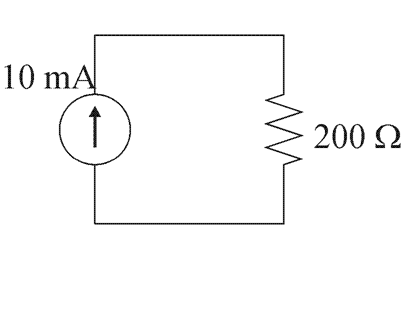Figure 1. A simple circuit.

In ECE 100 you learn that we may apply nodal analysis by introducing nodes which separate the circuit elements and grounding one of the nodes. This is shown in Figure 2.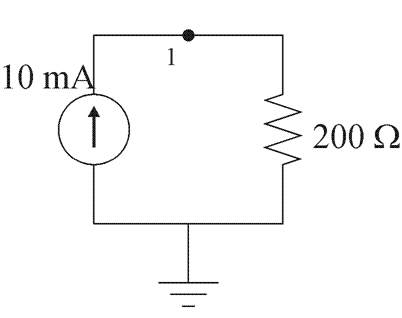Figure 2. Introducing a node.

Considering the one reminding node in this circuit, we apply Kirkhoff's current law (KCL), as is shown in Figure 3.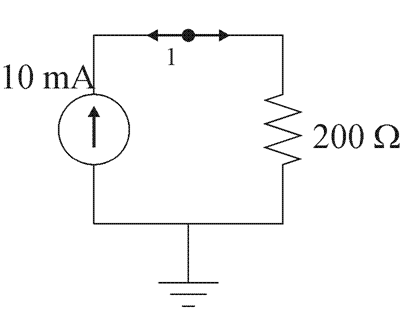Figure 3. Applying KCL to the circuit.

This gives us the equation:

−10 mA + 1/(200 Ω) (v1 − 0) = 0

This may be rearranged into the linear equation:

1/(200 Ω) v1 = 10 mA

which we may solve for v1 = 2 V.

# Nodal Analysis (More Interesting)

As a more interesting circuit, consider the circuit shown in Figure 4.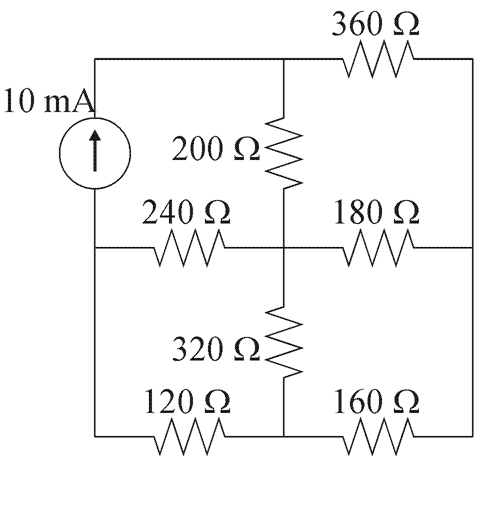Figure 4. A more interesting circuit.

Five nodes separate the circuit elements, one of which is grounded, as is shown in Figure 5.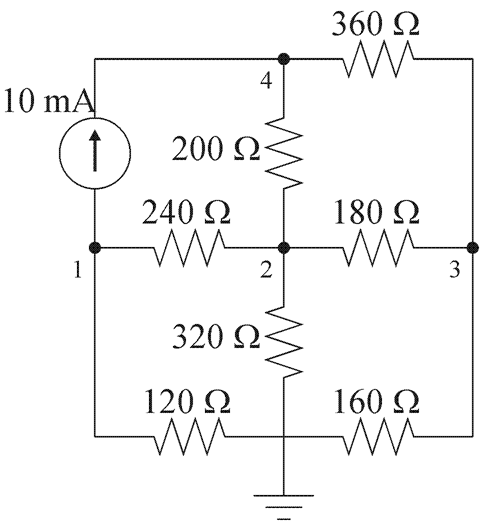Figure 5. Introducing nodes.

Then, applying KCL to Node 1, as is shown in Figure 6, we get the equation:

10 mA + 1/(120 Ω)(v1 − 0) + 1/(240 Ω)(v1v2) = 0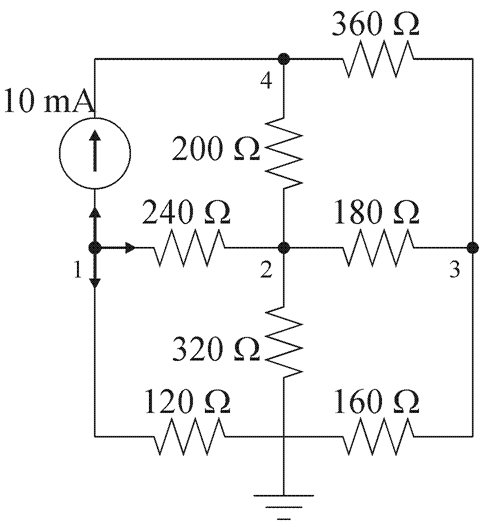Figure 6. Applying KCL to the circuit.

By removing the units and with appropriate scaling, if we apply this processing to the remaining nodes, we get the four equations:

0.01 + 1/120 (v1 − 0) + 1/240 (v1v2) = 0
1/320 (v2 − 0) + 1/240 (v2v1) + 1/180 (v2v3) + 1/200 (v2v4) = 0
1/160 (v3 − 0) + 1/180 (v3v2) + 1/360 (v3v4) = 0
-0.01 + 1/200 (v4v2) + 1/360 (v4v3) = 0

By expanding these out, we have a system of four linear equations in four unknowns, which may therefore be written as:

Solving this system gives us the voltage vector v = (-0.619, 0.544, 0.556, 1.83)T. Using this information, we can, for example, determine the voltage flowing between two nodes, the corresponding energy losses, etc.

Notice that the current only affects the right-hand vector, and therefore, we could change the currents, but continue to use the same conductance matrix. Thus, PLU decomposition would be more efficient than applying Gaussian elimination.

# Matlab

The follow Matlab code finds the PLU decomposition of the matrix M:

```M = [-6 -1 3.25 10.25; 12 2 1 0; 2.4 10.4 -1.8 2; 0 1 14.8 1.2]
[L U Pt] = lu( M )
```

Here, the lower triangular matrix is assigned to the first output, L, upper triangular matrix is assigned to the second, U, and the transpose of the permutation matrix is assigned to the third, Pt.

To do this manually, we would do the following:

```M = [-6 -1 3.25 10.25; 12 2 1 0; 2.4 10.4 -1.8 2; 0 1 14.8 1.2]
n = length( M );
L = zeros( n, n );
U = M;
Pt = eye( n, n );
for i=1:(n - 1)
% find the row with the maximum entry in the ith column on or below the diagonal
[tmp, j] = max( abs( U(i:n, i) ) ) ;
j = j + (i - 1);   % account for offset

if ( tmp == 0 )
error( 'the matrix is singular (non invertible)' );
end

% swap rows 'i' and 'j'
U ([i, j], :) = U ([j, i], :);
Pt([i, j], :) = Pt([j, i], :);
L ([i, j], :) = L ([j, i], :);

for j=(i + 1):n
s = -U(j, i)/U(i, i);          % calculate multiplier
U(j, :) = U(j, :) + s*U(i, :); % add the multiplier times the ith row onto the jth
L(j, i) = -s;                  % store the negated multiplier
end
end
L = L + eye( n, n );  % add on the identity matrix
```

To solve the system M x = b where

```b = [-0.75 22 -3.6 0.2]'
```

let Matlab use forward and backward substitution as follows:

```y = L \ (Pt * b)
x = U \ y
```

# Short Cut

If you simply wish to solve for x, just enter:

```x = M \ b
```

# Maple

The following commands in Maple finds the PLU decomposition of the given matrix M:

```M := <<-6, 12, 2.4, 0>|<-1, 2, 10.4, 1>|<3.25, 1, -1.8, 14.8>|<10.25, 0, 2, 1.2>>;
LinearAlgebra[LUDecomposition]( M );
b = <-0.75, 22, -3.6, 0.2>
LinearAlgebra[LinearSolve]( M, b );
```

For more help on either of these routines or on the LinearAlgebra package, enter:

```?LinearAlgebra
?LinearAlgebra,LUDecomposition
?LinearAlgebra,LinearSolve
```

# BLAS

The basic linear algebra subroutines (BLASs) for performing a PLU decomposition together with solving the result are the two functions:

```  int gsl_linalg_LU_decomp( gsl_matrix *M, gsl_permutation *Pt, int );
int gsl_linalg_LU_solve( gsl_matrix *M, gsl_permutation *Pt, gsl_vector *b, gsl_vector *x );
```

An example of the use of these functions which finds the coefficients of the polynomial interpolating the points (1, sin(1)), (2, sin(2)), ..., (n, sin(n)) is provided here in both a version which performs the operations but also prints the matrices and vectors plu.print.c and a version which simply performs the operations plu.c.

To compile and execute this code, you must have the GNU Scientific Library installed on your system, either in cygwin or in Linux. The example below looks at how you compile this code in Cygwin:

```\$ gcc -Wall -I/usr/include plu.print.c -lgsl -lgslcblas -lm
\$ ./a.exe
```

You may view the output.

Reference: GNU Scientific Library, 13.1 LU Decomposition.